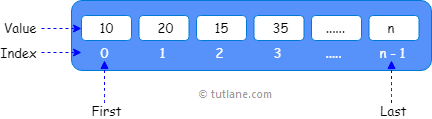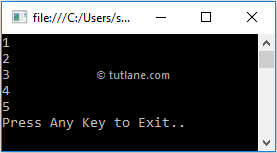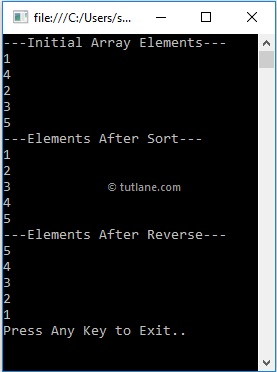# C# Arrays with Examples

In c#, Arrays are useful for storing multiple elements of the same data type at contiguous memory locations. It will store a fixed number of elements sequentially based on the predefined number of items.

In the previous chapter, we learned about variables in c#, which will help us hold a single value like int x = 10;. If we want to hold more than one value of the same data type, then an array came into the picture in c# to solve this problem.

An array can start storing the values from index 0. If we have an array with n elements, it will start storing the elements from index 0 to n-1.

Following is the pictorial representation of storing the multiple values of the same type in the c# array data structure.If you observe the above diagram, we are storing the values in an array starting from index 0, and it will continue to store the values based on the defined number of elements.

## C# Arrays Declaration

In c#, Arrays can be declared by specifying the type of elements followed by the square brackets [] as shown below.

type[] array_name;

Here, the type is nothing but a data type of elements to store in an array, and array_name represents an array's name.

For example, the following are the different ways of declaring an array with different data types in the c# programming language.

// Store only int values
int[] numbers;
//Store only string values
string[] names;
//Store only double values
double[] ranges;

If you observe the above examples, we declared arrays with the required data type based on our requirements.

In c#, the array elements can be of any type, and by default, the values of numeric array elements are set to zero, and the reference elements are set to null.

## C# Arrays Initialization

In c#, Arrays can be initialized by creating an instance of the array with a `new` keyword. Using a `new` keyword, we can declare and initialize an array simultaneously based on our requirements.

Following are the different ways of declaring and initializing array elements by using the `new` keyword in the c# programming language.

// Declaring and Initializing an array with a size of 5
int[] array = new int;
//Defining and assigning elements at the same time
int[] array2 = new int{1,2,3,4,5};
//Initialize with 5 elements will indicate the size of an array
int[] array3 = new int[] { 1, 2, 3, 4, 5 };
// Another way to initialize an array without the size
int[] array4 = { 1, 2, 3, 4, 5 };
// Declare an array without initialization
int[] array5;
array5 = new int[]{ 1, 2, 3, 4, 5 };

In the first statement, we declared and initialized an integer array with the size of 5 to allow an array to store 5 integer values. The array can contain elements from array to array.

In the second statement, we declared and initialized an array same as the first statement, and assigned values to each index followed by curly brackets { }.

In a third or fourth statement, while declaring, we initialized an array with values without specifying any size. Here, the size of an array can be determined by the number of elements, so the size initializer is not required if we assign elements during the initialization.

In c#, we can declare an array variable without initialization, but we must use the `new` keyword to assign an array to the variable.

In the fifth statement, we declared an array without initialization, and we used a `new` keyword to assign array values to the variable.

In c#, we can initialize array elements using index values after an array declaration. Following is an example of declaring and initializing array elements using individual index values in c#.

int[] array = new int;
array = 1;
array = 2;
array = 3;
array = 4;
array = 5;

If you observe the above example, we are initializing an array of elements individually using individual index values.

Generally, in c# initializing an array without size or assigning values to an array without a `new` operator will throw compile-time errors. For example:

// Error. Initialize an array without size
int[] array = new int[];
// Error. Initialize an array without a new keyword
int[] array1;
array1 = { 1, 2, 3, 4, 5 };

If you observe the above examples, we initialized an array without any size in the first statement. In the second statement, we declared and initialized array elements without using the new keyword. These two statements will throw compile-time errors in our c# applications.

## C# Accessing an Array Elements

In c#, we can access array elements using for loop or foreach loop or with particular index numbers.

Following is the code snippet of accessing array elements by using particular index numbers.

int[] array = new int { 1, 2, 3, 4, 5 };
int a = array; // It will return 2
int b = array; // It will return 5

If you observe the above code, we are trying to access an array of elements using index values in c#.

Following is the example of declaring, initializing, and accessing array elements with particular index numbers in the c# programming language.

using System;

namespace Tutlane
{
class Program
{
static void Main(string[] args)
{
int[] array = new int { 1, 2, 3, 4, 5 };
Console.WriteLine(array);
Console.WriteLine(array);
Console.WriteLine(array);
Console.WriteLine(array);
Console.WriteLine(array);
Console.WriteLine("Press Enter Key to Exit..");
}
}
}

If you observe the above example, we declared and initialized an array with 5 elements, and we are accessing an array of elements using index values.

When we execute the above c# program, we will get the result as below.If you observe the above result, we can access array elements using index numbers based on our requirements.

## C# Access Array Elements with For Loop

In c#, by using for loop we can iterate through array elements and access the values of an array with length property.

Following is the example of accessing array elements using for loop in the c# programming language.

using System;

namespace Tutlane
{
class Program
{
static void Main(string[] args)
{
int[] array = new int { 1, 2, 3, 4, 5 };
for (int i = 0; i < array.Length; i++)
{
Console.WriteLine(array[i]);
}
Console.WriteLine("Press Enter Key to Exit..");
}
}
}

If you observe the above example, we are looping through array elements with for loop to access array elements based on our requirements.

When we execute the above c# program, we will get the result below.If you observe the above result, we can loop through elements in an array with for loop and print array values based on our requirements.

## C# Access Array Elements with Foreach Loop

In c#, same as for loop, we can use the foreach loop to iterate through array elements and access the values of an array based on our requirements.

Following is the example of accessing array elements using a foreach loop in the c# programming language.

using System;

namespace Tutlane
{
class Program
{
static void Main(string[] args)
{
int[] array = new int { 1, 2, 3, 4, 5 };
foreach(int i in array)
{
Console.WriteLine(i);
}
Console.WriteLine("Press Enter Key to Exit..");
}
}
}

If you observe the above example, we are looping through array elements with a foreach loop to access array elements based on our requirements.

When we execute the above c# program, we will get the result as below.If you observe the above result, we can loop through elements in an array with a foreach loop and print array values based on our requirements.

This is how we can access array elements in the c# programming language based on our requirements.

## C# Array Types

In c#, we have different types of arrays available; those are

In this chapter, whatever the arrays we used, all are single-dimensional arrays. In the next chapters, we will learn about multi-dimensional and jagged arrays in a detailed manner.

## C# Array Class

In c#, we have a class called Array, and it will act as a base class for all the arrays in the common language runtime (CLR). The Array class provides methods for creating, manipulating, searching, and sorting arrays.

For example, by using the Sort or Copy methods of the Array class, we can sort the elements of an array and copy the elements of one array to another based on our requirements.

Following is the example of using an Array class to sort or filter or reverse array elements in the c# programming language.

using System;

namespace Tutlane
{
class Program
{
static void Main(string[] args)
{
int[] array = new int { 1, 4, 2, 3, 5 };
Console.WriteLine("---Initial Array Elements---");
foreach (int i in array)
{
Console.WriteLine(i);
}
Array.Sort(array);
Console.WriteLine("---Elements After Sort---");
foreach (int i in array)
{
Console.WriteLine(i);
}
Array.Reverse(array);
Console.WriteLine("---Elements After Reverse---");
foreach (int i in array)
{
Console.WriteLine(i);
}
Console.WriteLine("Press Enter Key to Exit..");
}
}
}

If you observe the above example, we are sorting and changing the order of array elements using the Sort and Reverse methods of an Array class.

When we execute the above c# program, we will get the result as below.If you observe the above result, we sorted the array elements and changed the order of array elements using the Array class based on our requirements.

This is how we can use the required methods of the Array class in our applications to implement the required functionality.